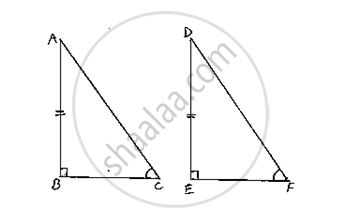Share
Notifications

View all notifications
Books Shortlist
Your shortlist is empty

# In Two Right Triangles One Side an Acute Angle of One Are Equal to the Corresponding Side and Angle of the Othe Prove that the Triangles Are Congruent. - CBSE Class 9 - Mathematics

Login
Create free account

Forgot password?
ConceptCongruence of Triangles

#### Question

In two right triangles one side an acute angle of one are equal to the corresponding side and angle of the othe Prove that the triangles are congruent.

#### Solution

Given that, in two right triangles one side and acute angle of one are equal to the corresponding side and angles of the other.

We have to prove that the triangles are congruent. Let us consider two right triangles such that

∠B = C = 90°         ........(1)

AB = DE                  ........(2)

∠C = F                 ........(3)

Now observe the two triangles ABC and DEF

∠.C = F             [From (3)]

∠B = E             [From (1)]

and AB = DE      [From (2)]

So, by AAS congruence criterion, we have

ΔABC ≅ ΔDEF

∴The two triangles are congruent Hence provedIs there an error in this question or solution?

#### APPEARS IN

Solution In Two Right Triangles One Side an Acute Angle of One Are Equal to the Corresponding Side and Angle of the Othe Prove that the Triangles Are Congruent. Concept: Congruence of Triangles.
S